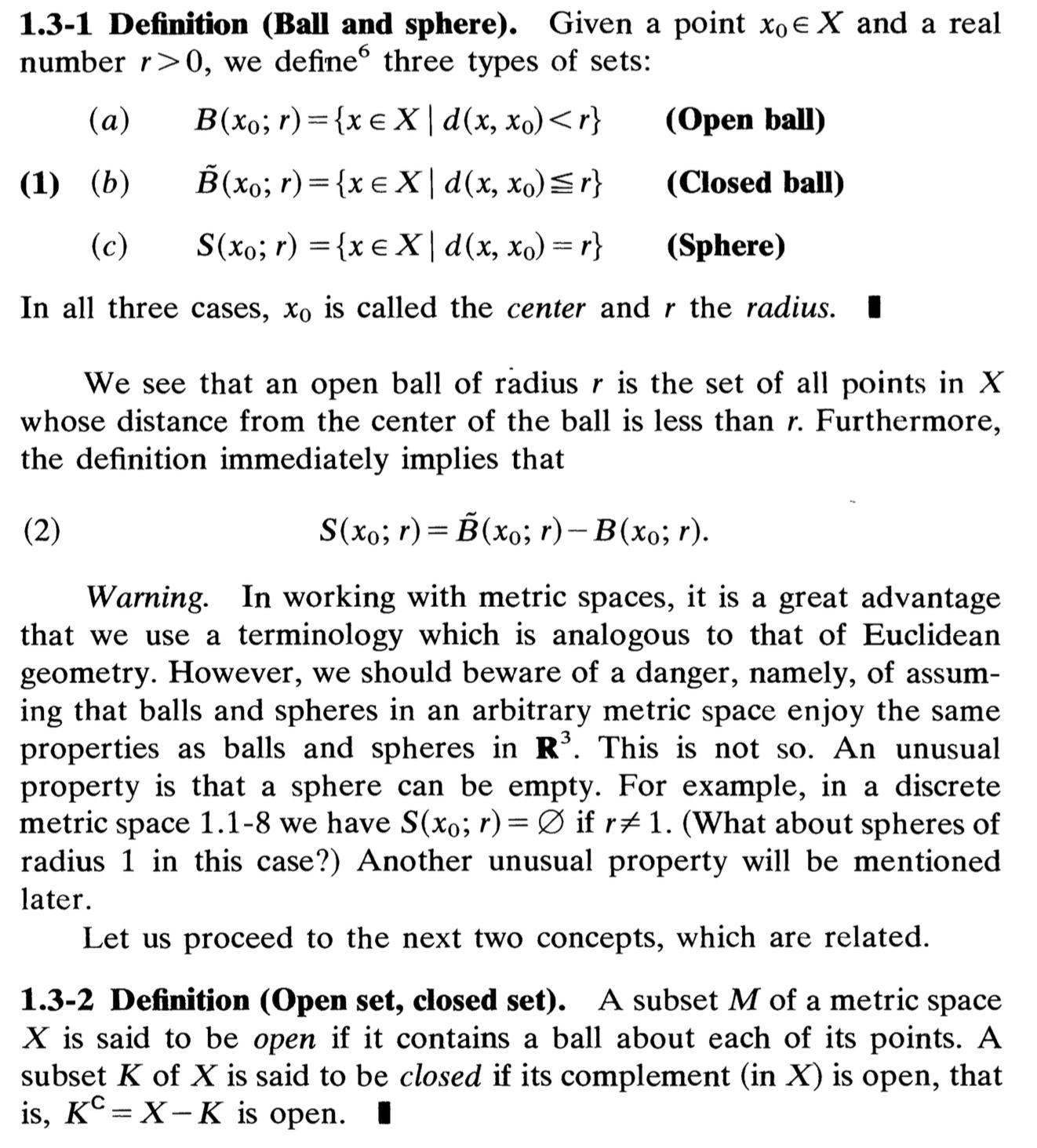## Wasserstein GAN (WGAN)

Generative Adversarial Network (GAN)The Minimal Mathematcis to understand Wasserstein GAN

2021년 12월 13일
·
0개의 댓글
·## The Mathematics for Wasserstein GAN

The subset $U$ of a metric space $M$ is said to be open if the subset $U$ contains $\\epsilon$-open neighborhood, defined as \$B(x, \\epsilon)=B\_{\\ep

2021년 12월 7일
·
0개의 댓글
·